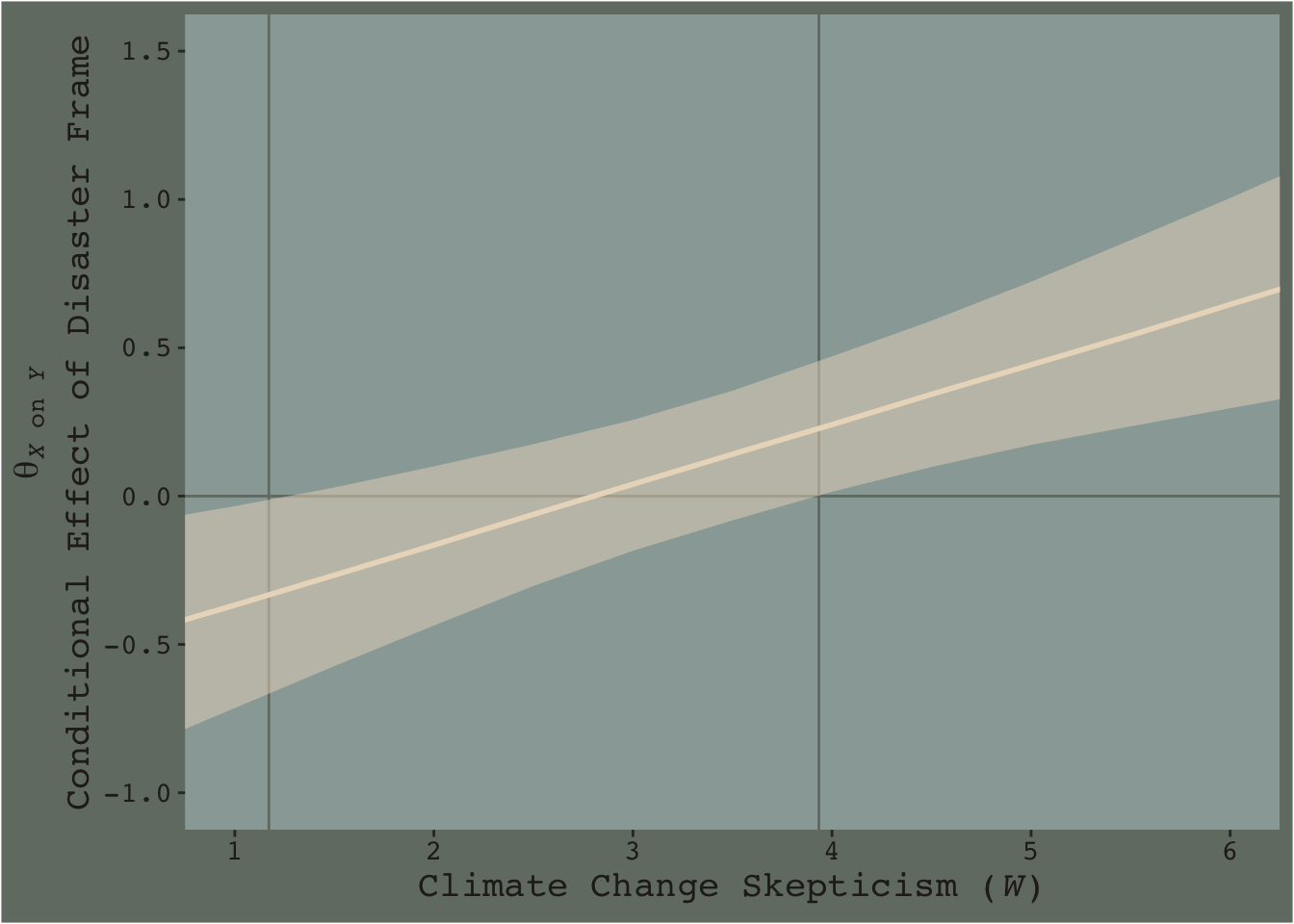## 7.6 Artificial categorization and subgroups

There are multiple ways to dichotomize the data by skeptic. A quick simple way is to use ifelse() to make a skeptic_hi dummy.

disaster <-
disaster %>%
mutate(skeptic_hi = ifelse(skeptic >= mean(skeptic), 1, 0))

With our dummy in hand, we’re ready to fit the two models.

model6_low <-
brm(data = disaster %>% filter(skeptic_hi == 0),
family = gaussian,
justify ~ 1 + frame,
chains = 4, cores = 4)

model6_high <-
update(model6_low, newdata = disaster %>% filter(skeptic_hi == 1),
chains = 4, cores = 4)

The coefficient summaries:

fixef(model6_low) %>% round(digits = 3)
##           Estimate Est.Error   Q2.5 Q97.5
## Intercept    2.626     0.099  2.431 2.824
## frame       -0.108     0.145 -0.390 0.173
fixef(model6_high) %>% round(digits = 3)
##           Estimate Est.Error  Q2.5 Q97.5
## Intercept    3.070     0.144 2.786 3.348
## frame        0.477     0.209 0.071 0.891

You can use fitted() to get the posterior means and other summaries for the two frame groups, by model.

fitted(model6_low,
newdata = tibble(frame = 0:1)) %>%
round(digits = 3)
##      Estimate Est.Error  Q2.5 Q97.5
## [1,]    2.626     0.099 2.431 2.824
## [2,]    2.518     0.105 2.309 2.724
fitted(model6_high,
newdata = tibble(frame = 0:1)) %>%
round(digits = 3)
##      Estimate Est.Error  Q2.5 Q97.5
## [1,]    3.070     0.144 2.786 3.348
## [2,]    3.548     0.149 3.256 3.836

Do note that though brms ‘Est.Error’ is the posterior $$SD$$ for the coefficient, it is not the same thing as descriptive statistic $$SD$$ of a subset of the data. Thus, although our ‘Estimates’ correspond nicely to the mean values Hayes reported in the middle of page 264, his $$SD$$s will not match up with our ‘Est.Error’ values, and nor should they.

Anyway, our results don’t yield $$t$$-tests. But you don’t need those anyway. We’re working within the regression paradigm! But if you’re really interested in the sub-model-implied differences between the two levels of frame by skeptic_hi subgroup, all you need is the frame coefficient of model6_low and model6_high. Here we’ll use bind_rows() to combine their posterior samples and then plot.

posterior_samples(model6_low) %>%
select(b_frame) %>%
bind_rows(
posterior_samples(model6_high) %>%
select(b_frame)
) %>%
mutate(model = rep(c("model6_low", "model6_high"), each = 4000)) %>%

ggplot(aes(x = b_frame, fill = model)) +
geom_density(size = 0, alpha = .8) +
scale_fill_manual(NULL,
values = dutchmasters\$little_street[c(1, 9)] %>% as.character()) +
scale_y_continuous(NULL, breaks = NULL) +
labs(subtitle = "The difference score distributions between frame levels,\ncolor coded by mean-split skeptic",
x = NULL) +
theme_07As within the frequentist paradigm, please don’t mean split as a Bayesian. When possible, use all available data and use the regression formula to model theoretically-meaningful variables in your analyses.